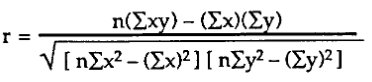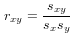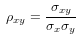# Correlation coefficient - Correlation & Regression, Business Mathematics & Statistics Notes | Study Business Mathematics and Statistics - B Com

## B Com: Correlation coefficient - Correlation & Regression, Business Mathematics & Statistics Notes | Study Business Mathematics and Statistics - B Com

The document Correlation coefficient - Correlation & Regression, Business Mathematics & Statistics Notes | Study Business Mathematics and Statistics - B Com is a part of the B Com Course Business Mathematics and Statistics.
All you need of B Com at this link: B Com

A correlation coefficient is a numerical measure of some type of correlation, meaning a statistical relationship between two variables. The variables may be two columns of a given data set of observations, often called a sample, or two components of a multivariate random variable with a known distribution.
Several types of correlation coefficient exist, each with their own definition and own range of usability and characteristics. They all assume values in the range from −1 to +1, where +1 indicates the strongest possible agreement and −1 the strongest possible disagreement. As tools of analysis, correlation coefficients present certain problems, including the propensity of some types to be distorted by outliers and the possibility of incorrectly being used to infer a causal relationship between the variables.

Types of correlation coefficient formulas.
There are several types of correlation coefficient formulas.
One of the most commonly used formulas in stats is Pearson’s correlation coefficient formula. If you’re taking a basic stats class, this is the one you’ll probably use:Two other formulas are commonly used: the sample correlation coefficient and the population correlation coefficient.

Sample correlation coefficientSx and sy are the sample standard deviations, and sxy is the sample covariance.

Population correlation coefficientThe population correlation coefficient uses σx and σy as the population standard deviations, and σxy as the population covariance.

What is Pearson Correlation?
Correlation between sets of data is a measure of how well they are related. The most common measure of correlation in stats is the Pearson Correlation. The full name is the Pearson Product Moment Correlation (PPMC). It shows the linear relationship between two sets of data. In simple terms, it answers the question, Can I draw a line graph to represent the data? Two letters are used to represent the Pearson correlation: Greek letter rho (ρ) for a population and the letter “r” for a sample.

Potential problems with Pearson correlation.
The PPMC is not able to tell the difference between dependent variables and independent variables. For example, if you are trying to find the correlation between a high calorie diet and diabetes, you might find a high correlation of .8. However, you could also get the same result with the variables switched around. In other words, you could say that diabetes causes a high calorie diet. That obviously makes no sense. Therefore, as a researcher you have to be aware of the data you are plugging in. In addition, the PPMC will not give you any information about the slope of the line; it only tells you whether there is a relationship.

The document Correlation coefficient - Correlation & Regression, Business Mathematics & Statistics Notes | Study Business Mathematics and Statistics - B Com is a part of the B Com Course Business Mathematics and Statistics.
All you need of B Com at this link: B ComUse Code STAYHOME200 and get INR 200 additional OFF

122 videos|142 docs

Track your progress, build streaks, highlight & save important lessons and more!

,

,

,

,

,

,

,

,

,

,

,

,

,

,

,

,

,

,

,

,

,

,

,

,

;